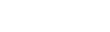# == could be used in Strings if we want to know whether both the objects are same or different, whereas .equals() could be used if we only want to know if the value is same or not.4 months ago | 3 min read
Follow# Understanding the difference

In Java, we know that for checking if the two values are equal or not, we use ==.

For example, if we execute the following code,

``public static void main(String args[]){        int a=5;        int b=5;        int c=7;        System.out.println(a==b);        System.out.println(a==c);}``

We will get the output of the first one as true and second one as false, which is quite obvious.

But what if we use == for comparing two strings..?

Let's see!

``public static void main(String args[]) {      //case 1      String first = new String("Hello");      String second = new String("Hello");      //case 2      String a = "People";      String b = "People";      System.out.println(first == second);      System.out.println(a==b);    }``

What do you think, what will be the answer?

Well...

This is the output. Now you might be wondering that why did the first output come as false although both the values are same, i.e. "Hello". And also, the second output is true. Why this sorcery?

# Reason behind the difference

Before getting to the main point, here are few things that we need to know:

## Some Important Concepts

1. Stack and Heap memory: There are two types of memory used in Java - a. Stack Memory and b. Heap Memory.

Stack memory stores the primitive data types and the address/reference of any objects.

Heap memory stores object value.

2. String pool: For storing the string values, a separate memory structure is present in the heap. If the value of two strings is same, no new space is allocated instead both the references point towards the same object in the string pool.

3. new Keyword: new keyword allocates memory space, i.e. it will create a different space for the object created.

Refer to the image.

== checks if the reference variables in the stack memory are pointing to the same object in the heap memory or not.

Therefore, in the case 2 of the above code, the output was true as String a and String b were pointing to the same object in the heap memory in the string pool.

Whereas in case 1, String first and String second are pointing towards different object, since there are two different objects created due the the new keyword, although their value is same. And thus returns false.

## .equals() function

So how to check if the value of the strings is same or not?

For this, java provides us .equals(). The .equals() checks whether the value of the strings are equal or not irrespective of where the reference variable is pointing to.

Let's consider this code:

``public static void main(String args[]) {      String first = new String("Hello");      String second = new String("Hello");            System.out.println(first == second);      System.out.println(first.equals(second));    }``

Here, we can see that .equals() returned true as the value is same irrespective of the fact that they are different objects.

# Visualizing the difference

Let's assume there is a basket of apples at David's house and a basket of apples at Rita's house.

Now if the question is asked, are they equal? There are two probabilities of answer.

Case 1: If == is used, then the answer will be false, i.e. NO, both the objects are different because one is a basket of apples at David's house and the other is at Rita's house. Since both of them are separate objects at different place, both are treated as different objects.

Case 2: If .equals() is used, then the answer will be true, i.e. YES, both the objects are same, i.e. they are apples, doesn't matter whether those are at David's house or Rita's. Since the value is same(apples), they are equal.

# Conclusion:

== could be used in Strings if we want to know whether both the objects are same or different, whereas .equals() could be used if we only want to know if the value is same or not.

In strings, .equals() is preferred because generally we just need to check whether the value is same or not irrespective of whether the reference variables are pointing to the same object or not.

Thanks for reading! I hope I have been successful in explaining the difference in the simplest way possible. Feel free to like [it motivates me:)] and share with your friends if you found this article helpful.

Happy learning!

UpvoteCreated by

Follow

Active Learner and Content Writer

I am sophomore, pursuing bachelors in Computer Science and Engineering who loves to learn new things and share with others. I share all my learnings through blogs and twitter threads.Post

Upvote

Downvote

Comment

Bookmark

Share

Related Articles